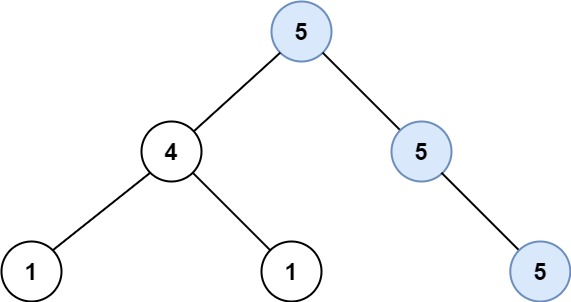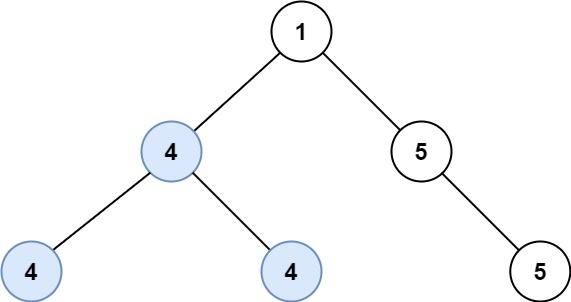## 687. Longest Univalue Path

Given the `root` of a binary tree, return the length of the longest path, where each node in the path has the same value. This path may or may not pass through the root.

The length of the path between two nodes is represented by the number of edges between them.

Example 1:```Input: root = [5,4,5,1,1,5]
Output: 2
```

Example 2:```Input: root = [1,4,5,4,4,5]
Output: 2
```

Constraints:

• The number of nodes in the tree is in the range `[0, 104]`.
• `-1000 <= Node.val <= 1000`
• The depth of the tree will not exceed `1000`.

## Rust Solution

``````struct Solution;
use rustgym_util::*;

trait LongestUnivaluePath {
fn longest_univalue_path(&self, max: &mut i32, parent_val: i32) -> i32;
}

fn longest_univalue_path(&self, max: &mut i32, parent_val: i32) -> i32 {
if let Some(node) = self {
let node = node.borrow();
let left = Self::longest_univalue_path(&node.left, max, node.val);
let right = Self::longest_univalue_path(&node.right, max, node.val);
*max = i32::max(left + right, *max);
if parent_val == node.val {
i32::max(left, right) + 1
} else {
0
}
} else {
0
}
}
}

impl Solution {
fn longest_univalue_path(root: TreeLink) -> i32 {
let mut max = 0;
root.longest_univalue_path(&mut max, 0);
max
}
}

#[test]
fn test() {
let root = tree!(1, tree!(4, tree!(4), tree!(4)), tree!(5, None, tree!(5)));
assert_eq!(Solution::longest_univalue_path(root), 2);
let root = tree!(3, tree!(3), None);
assert_eq!(Solution::longest_univalue_path(root), 1);
}
``````

Having problems with this solution? Click here to submit an issue on github.